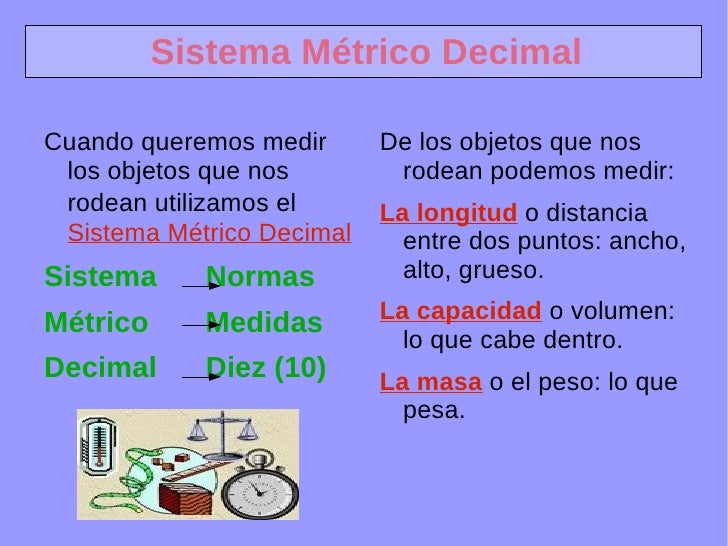Successfully reported this slideshow.
We use your LinkedIn profile and activity data to personalize ads and to show you more relevant ads. You can change your ad preferences anytime.Upcoming SlideShare
Loading in …5
×

# Sistema Metrico

54,272 views

Published on

Es una presentación para OpenOffice que puede servir para explicar el paso de unidades de longitud, capacidad y masa en el sistema métrico decimal, bien en la P.D.I. o bien en cada portátil del alumnado.

Published in: Education
• Full Name
Comment goes here.

Are you sure you want to Yes No
Your message goes here• Be the first to comment

### Sistema Metrico

1. 1. Sistema Métrico Decimal <ul><li>Cuando queremos medir los objetos que nos rodean utilizamos el Sistema Métrico Decimal
2. 2. Sistema Normas
3. 3. Métrico Medidas
4. 4. Decimal Diez (10) </li></ul><ul><li>De los objetos que nos rodean podemos medir:
5. 5. La longitud o distancia entre dos puntos: ancho, alto, grueso.
6. 6. La capacidad o volumen: lo que cabe dentro.
7. 7. La masa o el peso: lo que pesa. </li></ul>
8. 8. Sistema Métrico Decimal <ul>Longitudes el metro Capacidades el litro Masas el gramo </ul>MÚLTIPLOS Unidad SUBMÚLTIPLOS Kilómetro Hectómetro Decámetro Metro decímetro centímetro milímetro Kilólitro Hectólitro Decálitro Litro decílitro centílitro milílitro Kilógramo Hectógramo Decágramo Gramo decígramo centígramo milígramo
9. 9. Sistema Métrico Decimal Kilómetro Hectómetro Decámetro Metro decímetro centímetro milímetro Kilólitro Hectólitro Decálitro Litro decílitro centílitro milílitro Kilógramo Hectógramo Decágramo Gramo decígramo centígramo milígramo Para transformar una medida de una unidad a otra hay que tener en cuenta que van de 10 en 10. Esto quiere decir que 1 unidad de una magnitud equivale a 10 unidades de la que está a su derecha. O lo que es lo mismo 1 unidad equivale a 1 décima (1 décima) de la que está a su izquierda. x10 x10 x10 x10 x10 x10 :10 :10 :10 :10 :10 :10
10. 10. Sistema Métrico Decimal: La longitud Kilómetro (Km.) Hectómetro (Hm.) Kilómetro (Km.) Decámetro (dam.) Metro (m.) decímetro (dm.) centímetro (cm.) milímetro (mm.) 1 kilómetro = 10 hectómetros 1 metro = 10 decímetros 1 centímetro = 0,1 decímetros 1 metro = 0,1 decámetros
11. 11. Sistema Métrico Decimal: La longitud Kilómetro (Km.) Hectómetro (Hm.) Kilómetro (Km.) Decámetro (dam.) Metro (m.) decímetro (dm.) centímetro (cm.) milímetro (mm.) 1 kilómetro = 100 decámetros 1 metro = 100 centímetros 1 milímetro = 0,01 decímetros 1 metro = 0,01 hectómetros
12. 12. Sistema Métrico Decimal: La longitud Kilómetro (Km.) Hectómetro (Hm.) Kilómetro (Km.) Decámetro (dam.) Metro (m.) decímetro (dm.) centímetro (cm.) milímetro (mm.) 1 kilómetro = 1.000 metros 1 metro = 1.000 milímetros 1 milímetro = 0,001 metro 1 metro = 0,001 kilómetro
13. 13. Sistema Métrico Decimal: La longitud Kilómetro (Km.) Hectómetro (Hm.) Kilómetro (Km.) Decámetro (dam.) Metro (m.) decímetro (dm.) centímetro (cm.) milímetro (mm.) 1 km = 10 hm 1 km = 100 dam 1 km = 1.000 m 1 km = 10.000 dm 1 km = 100.000 cm 1 km = 1.000.000 mm Observa que al bajar a una unidad más pequeña el número es 10 veces mayor en cada escalón
14. 14. Sistema Métrico Decimal: La longitud Kilómetro (Km.) Hectómetro (Hm.) Kilómetro (Km.) Decámetro (dam.) Metro (m.) decímetro (dm.) centímetro (cm.) milímetro (mm.) 1 mm = 0,000001 km 1 mm = 0,00001 hm 1 mm = 0,0001 dam 1 mm = 0,001 m 1 mm = 0,01 dm 1 mm = 0,1 cm Observa que al subir a unidad más grande el número es 10 veces menor en cada escalón
15. 15. Sistema Métrico Decimal: La longitud Kilómetro (Km.) Hectómetro (Hm.) Kilómetro (Km.) Decámetro (dam.) Metro (m.) decímetro (dm.) centímetro (cm.) milímetro (mm.) Para bajar se multiplica por 10, 100, 1.000, etc. X Para subir se divide entre 10, 100, 1.000, etc. :
16. 16. Sistema Métrico Decimal: La longitud Kilómetro (Km.) Hectómetro (Hm.) Kilómetro (Km.) Decámetro (dam.) Metro (m.) decímetro (dm.) centímetro (cm.) milímetro (mm.) 4 hm = 400 m ---> (Se multiplica 4 x 100) X : 1,5 km = 1.500 m ---> (Se multiplica 1,5 x 1000) 1,75 m = 175 cm ---> (Se multiplica 1,75 x 100) 75 m = 7,5 dam ---> (Se divide 75 : 10) 700 cm = 7 m ---> (Se divide 700 : 100) 5 m = 0,005 km ---> (Se divide 5 : 1000)
17. 17. Recuerda Kilómetro (Km.) Hectómetro (Hm.) Kilómetro (Km.) Decámetro (dam.) Metro (m.) decímetro (dm.) centímetro (cm.) milímetro (mm.) X 1.000 X 100 : 1.000 X 10 : 10 : 100
18. 18. Sistema Métrico Decimal: La longitud